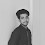# URI - BEECROWD - BEE Online Judge Solution  1018 | Banknotes - URI - BEECROWD - BEE 1018 Solution in C,C++,Python

In this problem you have to read an integer value and calculate the smallest possible number of banknotes in which the value may be decomposed. The possible banknotes are 100, 50, 20, 10, 5, 2 e 1. Print the read value and the list of banknotes.

## Input

The input file contains an integer value (0 < < 1000000).

## Output

Print the read number and the minimum quantity of each necessary banknotes in Portuguese language, as the given example. Do not forget to print the end of line after each line, otherwise you will receive “Presentation Error”.

 Input Sample Output Sample 576 5765 nota(s) de R\$ 100,001 nota(s) de R\$ 50,001 nota(s) de R\$ 20,000 nota(s) de R\$ 10,001 nota(s) de R\$ 5,000 nota(s) de R\$ 2,001 nota(s) de R\$ 1,00

### URI Online Judge Solution  1018 | Banknotes - URI 1018 Solution in C,C++,Python::

URI Problem 1018 Solution in C :

 URI Online Judge 1018 Solve  in C :
``` #include <stdio.h>

int main(){
int notes, aux;

scanf("%d", &notes);

printf("%d\n", notes);
printf("%d nota(s) de R\$ 100,00\n", notes/100);
aux = (notes%100);

printf("%d nota(s) de R\$ 50,00\n", aux/50);
aux = (aux%50);

printf("%d nota(s) de R\$ 20,00\n", aux/20);
aux = (aux%20);

printf("%d nota(s) de R\$ 10,00\n", aux/10);
aux = (aux%10);

printf("%d nota(s) de R\$ 5,00\n", aux/5);
aux = (aux%5);

printf("%d nota(s) de R\$ 2,00\n", aux/2);
aux = (aux%2);

printf("%d nota(s) de R\$ 1,00\n", aux/1);
return 0;
}```

### 3 Responses to URI - BEECROWD - BEE 1018 | Banknotes Solution in C,C++,Python

1.Thanks Nice Thinking

2.3.can anyone tell me why this code is not accepted ?

#include
int main()
{
int notes[] = {100,50,20,10,5,2,1};
int A,B;
//bool C = true;
scanf("%d",&A);
B = A;
while(B!=0 && 0<A && A<1000000){

for(int i = 0; i < 7; i++){

printf("%d nota(s) de R\$ %d,00\n",(B/notes[i]),notes[i]);
B = B%notes[i];
}

}

return 0;
}